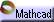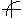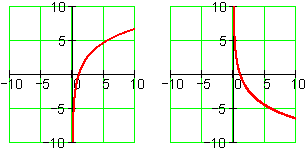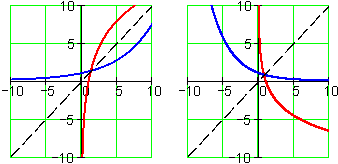Logarithmic Functions Click here to open an associated Mathcad worksheet:Graphic RepresentationLogarithmic graphs are asymptotic to the y-axis: In the long term, in one direction or the other, they become arbitrarily close to, but never touch, the y-axis.

They live entirely in the half plane to the right of the y-axis.

The curves pull away from the y-axis, increasing at a decreasing rate (less positive) or decreasing at an increasing rate (less negative).

Logarithmic graphs are reflections (mirror images) of exponential graphs. The "mirror" is the line  y = x :Only exponential functions  y = a b x  with  a > 0  are reflections of logarithmic functions.

Every logarithmic graph has an x-intercept at  (1, 0) . Regardless of  a  and  b , a logb(1) = 0 .

The above graphs show just a few of the members of the family of logarithmic functions. To see how these and other logarithmic graphs can be created by tuning the family's parameters, see the associated worksheet.